UPSC  >  Introduction: Number System

# Introduction: Number System - Notes | Study CSAT Preparation for UPSC CSE - UPSC

 Table of contentsIntroductionUnderstanding NumbersClassification of NumbersDivisibility TestProperties of NumberNumber System QuestionsAbsolute Value of a Number1 Crore+ students have signed up on EduRev. Have you?

Introduction

• Numbers form an integral part of our lives. In this lesson, we will learn about the different types of numbers and the different categories under which they fall.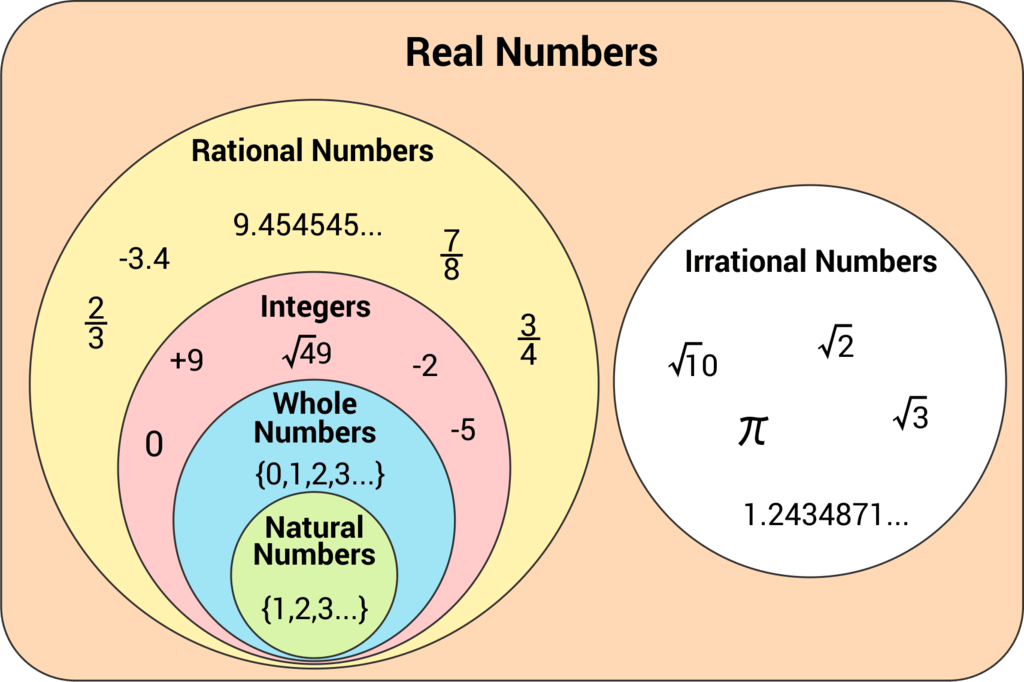Classification of Numbers
• The concepts discussed in this lecture will be your first step towards a general understanding of the mathematics requirements to clear MBA entrance exams.
• As we proceed with this lecture, you will realize that you have already learnt many of the concepts, included in this lesson, in school. This would further help build confidence in you.
• Although Number theory is important in the context of all the MBA entrance exams, it gains all the more important for the students aiming for success in the CAT.

Understanding Numbers

• A measurement carried out, of any quantity, leads to a meaningful value called the Number.
• This value may be positive or negative depending on the direction of the measurement and can be represented on the number line.

Natural Numbers (N)

• The numbers 1, 2, 3, 4, 5…are known as natural numbers. The set of natural numbers is denoted by N.
• Hence, N = {1, 2, 3, 4…}. The natural numbers are further divided as even, odd, prime etc.
• Whole Numbers (W): All natural numbers together with ‘0’ are collectively called whole numbers. The set of whole numbers is denoted by W, and W = {0, 1, 2, 3, ……}

Integers (Z)

• The set including all whole numbers and their negatives is called a set of integers. It is denoted by Z, and Z = {- ∞, … - 3, - 2, - 1, 0, 1, 2, 3, ……. ∞}.
• They are further classified into Negative integers, Neutral integers and positive integers.
(a) Negative integers (Z-)
All integers lesser than Zero is called negative integers.
Z− = {- 1, - 2, - 3…- ∞ }
(b) Neutral integers (Z0)
Zero is the only integer that is neither negative nor positive and it is called a neutral integer.
(c) Positive integers (Z+)
All integers greater than zero are called positive integers.
Z+ = {1, 2, 3, …….., ∞ }.

Classification of Numbers

Even Numbers

All numbers divisible by 2 are called even numbers.

Example: 2, 4, 6, 8, 10.…

Even numbers can be expressed in the form 2n, where n is an integer. Thus 0, - 2, − 6, etc. are also even numbers.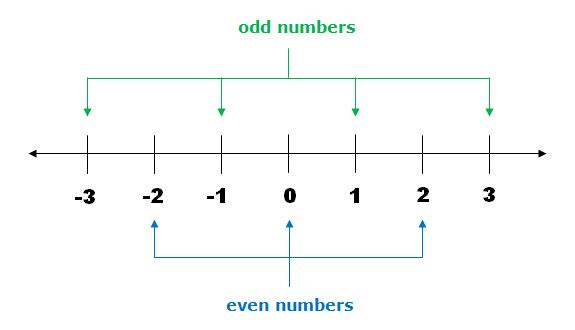Even and odd numbers

Odd Numbers

All numbers not divisible by 2 are called odd numbers.

Odd numbers can be expressed in the form (2n + 1) where n is an integer. Thus - 1, − 3, − 9 etc. are all odd numbers.

Example: 1, 3, 5, 7, 9…

Prime Numbers

A natural number that has no other factors besides itself and unity is a prime number.

Examples: 2, 3, 5, 7, 11, 13, 17, 19 …

• A prime number greater than 3, when divided by 6 leaves either 1 or 5 as the remainder.
• Hence, a prime number can be expressed in the form of 6K ± 1.
• But the converse of this observation is not true, that a number leaving a remainder of 1 or 5 when divided by 6 is not necessarily a prime number.
Example: 25, 35 etc

Tip: If a number has no prime factor equal to or less than its square root, then the number is a prime number.

Must Know
1 to 25 ⇒ 9 prime
1 to 50 ⇒ 15 prime
1 to 100 ⇒ 25 prime
1 to 200 ⇒ 45 prime

Example: If a, a + 2, a + 4 are consecutive prime numbers. Then how many solutions ‘a’ can have?
(a) One
(b) Two
(c) Three
(d) More than three

• No even value of ‘a’ satisfies this. So ‘a’ should be odd.
• But out of three consecutive odd numbers, at least one number is a multiple of 3.
• So, the only possibility is a = 3 and the numbers are 3, 5, 7.

Composite Numbers

A composite number has other factors besides itself and unity.

Example: 8, 72, 39, etc.

On the basis of this fact that a number with more than two factors is a composite, we have only 34 composites from 1 to 50 and 40 composites from 51 to 100.

Tip: 1 is neither a prime number nor a composite number.

Perfect Numbers

• A number is said to be a perfect number if the sum of ALL its factors excluding itself (but including 1) is equal to the number itself or the sum of all the possible factors of the number is equal to twice the number.
• Funda: If the factors of any perfect number other than 1 are written and reciprocal of them are added together, then the result is always unity.

Example: 6 is a perfect number because the factors of 6, i.e., 1, 2 and 3, add up to the number 6 itself.
Also, 1/6 + 1/3 + 1/2 = (1+ 2+3/6) = 6/8 = 1 (Unity)
Other examples of perfect numbers are 28, 496, 8128, etc.
There are 27 perfect numbers discovered so far.

Co-Prime Numbers

Two numbers are (prime or composite) said to be co-prime to one another, if they do not have any common factor other than 1.

Example: 35 & 12, since they both don’t have a common factor among them other than 1.

Tip: The HCF of Co-prime number is always 1.

Fractions

A fraction denotes the part or parts of a unit. Several types are:

• Common Fraction: Fractions whose denominator is not 10 or a multiple of it. Example: 2/3, 17/18
• Decimal Fraction: Fractions whose denominator is 10 or a multiple of 10.
• Proper Fraction: In this the numerator < denominator.
Example: 2/10, 6/7, 8/9 etc. Hence its value < 1.
• Improper Fraction: In these the numerator > denominator
Example: 10/2, 7/6, 8/7  etc. Hence its value > 1.
• Mixed Fractions: When an improper fraction is written as a whole number and proper fraction, it is called a mixed fraction.
Example: 7/3 can be written as 2 + 1/3 = 2(1/3)

Rational Numbers

• Rational Number is defined as the ratio of two integers, i.e. a number that can be represented by a fraction of the form p/q where p and q are integers and q ≠ 0.
• They also can be defined as the non-terminating recurring decimal numbers. Such as 3.3333…., 16.123123….. are all rational numbers as they can be expressed in the form p/q.

Examples: Finite decimal numbers, whole numbers, integers, fractions
i.e. 3/5, 16/9, 0.666...∞, 2/3, 7, 0 etc.

Irrational Numbers

• Any number which can not be represented in the form p/q where p and q are integers and q ≠ 0 is an irrational number.
• On the basis of non-terminating decimals, irrational numbers are non-terminating non-recurring decimals.
• Such as 3.4324546345……. is a non-terminating, non-repeating number.

Examples: π, √5, √7, e

Non-Terminating Decimal Numbers

• When we divide any number by another number, either we get a terminating number or a non - terminating number.

A non - terminating number on the basis of occurrence of digits after decimal can be classified as follows:

• Pure Recurring Decimals: A decimal in which all the figures after the decimal point repeat, is called a pure recurring decimal.
Examples: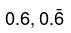are examples of pure recurring decimals.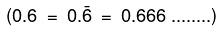• Mixed Recurring Decimals: A decimal in which some figures do not repeat and some of them are repeated is called a mixed recurring decimal.
Examples: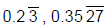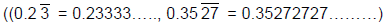• Non-Recurring Decimals: A decimal number in which the figure don’t repeat itself in any pattern is called non-terminating non-recurring decimals and are termed as irrational numbers.
• Converting Recurring Decimal as Fraction: All recurring decimals can be converted into fractions. Some of the common types can be 0.33…..,0.1232323…, 5.33…., 14.23636363…. etc.

(a) Pure Recurring to Fractions

Funda 1: If a number is of the form of 0. ababab……. then divide the repeating digits with as many 9’s as we have repeated digits.
Example: 0.363636...= 36/99 = 4/11

(b) Mixed Recurring to Fractions

Funda 2: If N = 0. abcbcbc…. Then N = abc - a/990 = Repeated & non Repeated digits - Non Repeated digits/ As many 9's as repeated digits followed by as many zero as non - repeated digits
Example: 0.25757...= 257 - 2/990 = 255/990 = 17/66

(c) Funda 3. If N = a. bcbc…. Then
Write N = a + 0. bcbc….Proceed as Funda 1
5.3636… = 5 + 0.3636… = 5 + 36/99 = 59/11

Divisibility Test

As the name suggests, divisibility tests or division rules in Maths help one to check whether a number is divisible by another number without the actual method of division. If a number is completely divisible by another number then the quotient will be a whole number and the remainder will be zero.

• Since every number is not completely divisible by every other number such numbers leave a remainder other than zero. These rules are certain ones, which help us to determine the actual divisor of a number just by considering the digits of the number.
• The division rules from 1 to 13 in Maths are explained here in detail with the solved examples.

Divisibility Rule of 1

• Every number is divisible by 1. The divisibility rule for 1 doesn’t have any condition. Any number divided by 1 will give the number itself, irrespective of how large the number is.
• Example: 3 is divisible by 1 and 3000 is also divisible by 1 completely.

Divisibility Rule of 2

• If a number is even or a number whose last digit is an even number i.e.  2,4,6,8 including 0, it is always completely divisible by 2.
• Example: 508 is an even number and is divisible by 2 but 509 is not an even number, hence it is not divisible by 2. The procedure to check whether 508 is divisible by 2 or not is as follows:
⇒ Consider the number 508
⇒ Just take the last digit 8 and divide it by 2
⇒ If the last digit 8 is divisible by 2 then the number 508 is also divisible by 2.

Divisibility Rules for 3

• Divisibility rule for 3 states that a number is completely divisible by 3 if the sum of its digits is divisible by 3.
• Example: Consider a number, 308. To check whether 308 is divisible by 3 or not, take sum of the digits (i.e. 3+0+8= 11). Now check whether the sum is divisible by 3 or not. If the sum is a multiple of 3, then the original number is also divisible by 3. Here, since 11 is not divisible by 3, 308 is also not divisible by 3.
• Similarly, 516 is divisible by 3 completely as the sum of its digits i.e. 5+1+6=12, is a multiple of 3.

Divisibility Rule of 4

• If the last two digits of a number are divisible by 4, then that number is a multiple of 4 and is divisible by 4 completely.
• Example: Take the number 2308. Consider the last two digits i.e.  08. As 08 is divisible by 4, the original number 2308 is also divisible by 4.

Divisibility Rule of 5

• Numbers, which last with digits, 0 or 5 are always divisible by 5.
• Example: 10, 10000, 10000005, 595, 396524850, etc.

Divisibility Rule of 6

• Numbers which are divisible by both 2 and 3 are divisible by 6. That is, if the last digit of the given number is even and the sum of its digits is a multiple of 3, then the given number is also a multiple of 6.
• Example: 630, the number is divisible by 2 as the last digit is 0.
The sum of digits is 6+3+0 = 9, which is also divisible by 3.
Hence, 630 is divisible by 6.

Divisibility Rules for 7

The rule for divisibility by 7 is a bit complicated which can be understood by the steps given below: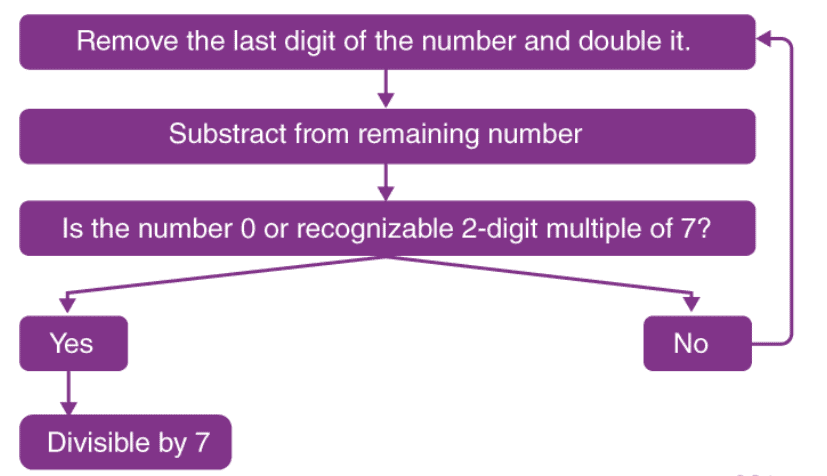Example: Is 1073 divisible by 7?

• From the rule stated remove 3 from the number and double it, which becomes 6.
• Remaining number becomes 107, so 107-6 = 101.
• Repeating the process one more time, we have 1 x 2 = 2.
• Remaining number 10 – 2 = 8.
• As 8 is not divisible by 7, hence the number 1073 is not divisible by 7.

Divisibility Rule of 8

• If the last three digits of a number are divisible by 8, then the number is completely divisible by 8.
• Example: Take number 24344.
Consider the last two digits i.e. 344.
As 344 is divisible by 8, the original number 24344 is also divisible by 8.

Divisibility Rule of 9

• The rule for divisibility by 9 is similar to divisibility rule for 3. That is, if the sum of digits of the number is divisible by 9, then the number itself is divisible by 9.
• Example: Consider 78532, as the sum of its digits (7+8+5+3+2) is 25, which is not divisible by 9, hence 78532 is not divisible by 9.

Divisibility Rule of 10

• Divisibility rule for 10 states that any number whose last digit is 0, is divisible by 10.
• Example: 10, 20, 30, 1000, 5000, 60000, etc.

Divisibility Rules for 11

If the difference of the sum of alternative digits of a number is divisible by 11, then that number is divisible by 11 completely.

In order to check whether a number like 2143 is divisible by 11, below is the following procedure:

• Group the alternative digits i.e. digits which are in odd places together and digits in even places together. Here 24 and 13 are two groups.
• Take the sum of the digits of each group i.e. 2+4=6 and 1+3= 4
• Now find the difference of the sums; 6-4=2
• If the difference is divisible by 11, then the original number is also divisible by 11. Here 2 is the difference which is not divisible by 11.
• Therefore, 2143 is not divisible by 11.

Divisibility Rule of 12

• If the number is divisible by both 3 and 4, then the number is divisible by 12 exactly.
• Example: 5864
⇒ Sum of the digits = 5 + 8 + 6 + 4 = 23 (not a multiple of 3)
⇒ Last two digits = 64 (divisible by 4)
⇒ The given number 5846 is divisible by 4 but not by 3; hence, it is not divisible by 12.

Divisibility Rules for 13

• For any given number, to check if it is divisible by 13, we have to add four times of the last digit of the number to the remaining number and repeat the process until you get a two-digit number.
• Now check if that two-digit number is divisible by 13 or not. If it is divisible, then the given number is divisible by 13.
• Example: 2795 ⇒ 279 + (5 x 4)
⇒ 279 + (20) ⇒ 299 ⇒ 29 + (9 x 4) = 29 + 36 = 65
Number 65 is divisible by 13, 13 x 5 = 65.

Properties of Number

• Every number has the same unit’s digit at its fifth power as it has at its first power. Thus the standard method that can be followed is to divide the power given by 4, find the remaining power and check the unit’s digit in that number. This shortcut can be applied because you will always get the same unit’s digit as otherwise.
• For calculating the number of zeroes at the end of the factorial of a number, you should divide the number by 5, the quotient obtained is again divided by 5 and so on till the last quotient obtained is smaller than 5. The sum of all the quotients is the number of 5s, which then becomes the number of zeroes in the given number.
• The digital root of a number is the sum of the digits, over and over again, till it becomes a single-digit number. For example, the digital root of 87984 will be 8 + 7 + 9 + 8 + 4 ⇒ 36 = 3 + 6 ⇒ 9.
• When the concept of Euler number is used and the dividend and divisor happen to be co-prime, the remainder questions become very easy.
• The product of 3 consecutive natural numbers is divisible by 6.
• For example, Let n,n+1,n+2 be three consecutive natural numbers and P (n) be their product.
• Then,P(n)=n(n+1)(n+2)
• We have,
P(1)=1×2×3=6, which is divisible by 3 and 6.
P(2)=2×3×4=24, which is divisible by 3, 8 and 6.
P(3)=3×4×5=60, which is divisible by 3 and 6.
P(4)=4×5×6=120, which is divisible by 3, 8 and 6.
Hence, P(n) is divisible by 3 and 6 for all  n∈ N.
• The product of 3 consecutive natural numbers, the first of which is an even number is divisible by 24.
• The sum of a two-digit number and a number formed by reversing its digits is divisible by 11.

Example 1: 28 + 82 = 110, this is divisible by 11. At the same time, the difference between those numbers will be divisible by 9.

Example 2: 82 – 28 = 54, this is divisible by 9.

• ∑n = n(n+1)/2, ∑n is the sum of first n natural numbers.
• ∑n2 = n(n+1)(2n+1)/6, ∑n2 is the sum of first n perfect squares.
• ∑n3 = n2(n+1)2/4 = (∑n)2, ∑n3 is the sum of first n perfect cubes.
• xn + yn = (x + y) (xn-1 - xn-2.y + xn-3.y2 - ... +yn-1) when n is odd. Therefore, when n is odd, xn + yn is divisible by x + y.

Example: 33 + 23 = 35 and is divisible by 5, which is (3 + 2).

• xn - yn = (x + y) (xn-1 - xn-2.y + ... yn-1) when n is even. Therefore, when n is even, xn - yn is divisible by x + y. e.g. 72 - 32 = 40, is divisible by 10, which is (7+3).
• xn - yn = (x - y) (xn-1 + xn-2.y + ... yn-1) for both odd and even n. Therefore, xn - yn is divisible by x - y. For example: 94 - 24 = 6545, is divisible by 7, which is (9 – 2).

Number System QuestionsExample 1. N = (18n2 + 9n + 8)/n; where N belongs to integer. How many integral solutions of N are possible?

The given expression can be broken as: 18n2/n + 9n/n + 8/n. This gives us: 18n + 9 + 8/n.

• Now we can see that whatever the value of ‘n’, 18n + 9 will always give an integral value. Therefore, it now depends upon 8/n the only ⇒ n can have any integral value, which is a factor of 8.
• The integers, which will satisfy this condition are ±1, ±2, ±8, ±4. Thus, in total, n can take 8 values.

Example 2. N = 960. Find the total number of factors of N.

• The number of divisors of a composite number: If D is a composite number in form D = ap × bq × cr, where a, b, c are primes, then the no. of divisors of D, represented by n is given by n = (p+1)(q+1)(r +1).
• Following the same, after dividing 960 into prime factors: 26 × 31 × 51, we can calculate the total number of factors as (6+1). (1+1).(1+1) = 28.

Example 3. Find the unit digit of the following expression: (123)34 × (876)456 × (45)86.

• Whenever an even unit digit and a 5 at the unit digit are present, they will always give a 0 at the unit digit, no matter if any other number is present or not.
• Hence, with this approach, this question proves to be a sitter.

Example 4. What will be the number of zeroes at the end of the product of the first 100 natural numbers?

• In this kind of questions, you need to find the greatest power of 5, which can divide the product of the first 100 natural numbers.
• Remember, a multiple of 5 multiplied by any even number, gives you a zero. Now divide 100 by 5 and take 20 as the quotient. Then divide 20 (the quotient) by 5 and get the new quotient 4, which further cannot be divided by 5.
• The sum of all such quotient gives you the greatest power of 5, which can divide that number. The sum is 24 and this is the number of zeroes at the end of the product of the first 100 natural numbers.

Example 5. Which letter should replace the \$ in the number 2347\$98, so that it becomes a multiple of 9?

• As you know that if the sum of all the digits is divisible by 9, then the number is divisible by 9.
• Now sum of the given digits is 2 + 3 + 4 + 7 + 9 + 8 = 33 + \$. Now think the next multiple of 9 after 33 i.e. 36. This means you add 3 in this. The value of \$ is 3.

Example 6. In a party, there are 20 persons present. If each of them shakes hand with all the other persons, in total, how many handshakes will take place?

• Out of 20 persons, the first person will shake hand with 19 persons.
• The second person will shake hand with 18 persons (because he has already shaken hand with first person).
• The third person will shake hand with 17 persons and so on.
• The second last person shakes hand with only one person.
• And last will shake hand with none (because he has already shaken hand with all persons).
• In order to find the total number of handshakes, you have to add all the natural numbers from 1 to 19, i.e. ∑ 19. ∑19 = 19 x 20/2 = 190 handshakes.

Example 7. What is the remainder when 2354789341 is divided by 11?

• Odd place digit sum (O) = 1 + 3 + 8 + 4 + 3 = 19.
• Even place digits sum (E) = 4 + 9 + 7 + 5 + 2 = 27.
• Difference (D) = 19 - 27 = - 8
• Remainder = 11 - 8 = 3.

Tip: ⇒ When any number with even number of digits is added to its reverse, the sum is always divisible by 11. e.g. 2341 + 1432 = 3773, which is divisible by 11.

⇒ Any number written 6 times consecutively will be divisible by 7 and 13.

Example 8. If 567P55Q is divisible by 88; Find the value of P + Q.
(a) 11
(b) 12
(c) 5
(d) 6
(e) 10

• The number is divisible by 8 means; the number formed by the last 3 digits should be divisible by 8, which are 55 Q. Only Q = 2 satisfies this.
• From the divisibility rule of 11, (2 + 5 + 7 + 5) - (5 + P + 6) is divisible by 11. So 8-P is divisible by 11.
• If P= 8, then only it is possible. So P = 8 and Q = 2.
• So, P + Q = 10.

Example 9. If the first 100 natural numbers are written side by side to form a big number and it is divided by 8. What will be the remainder?

(a) 1
(b) 2
(c) 4

(d) 7
(e) Cannot be determined

• The number is 1234…..9899100.
• According to the divisibility rule of 8, we will check only the last 3 digits.
• If 100 is divided by 8, the remainder is 4.

Example 10. What will be the remainder when 4444……..44 times is divided by 7?
(a) 1
(b) 2
(c) 5
(d) 6
(e) 0

• If 4 is divided by 7, the remainder is 4.
• If 44 is divided by 7, the remainder is 2.
• If 444 is divided by 7, the remainder is 3.
• By checking like this, we come to know that 444444 is exactly divisible by 7.
• So if we take six 4’s, it is exactly divisible by 7.
• Similarly, twelve 4’s is also exactly divisible by 4 and 42 4’s will be exactly divisible by 7. So out of 44, the remaining two 4,s will give a remainder of 2.

Absolute Value of a Number

• The modulus of a number is the absolute value of the number or we can say the distance of the number from the origin. The absolute value of a number is defined as:
|a| = a, if a ≥ 0
= - a, if a ≤ 0
|a| is read as MODULUS of a
• Example: |79| = 79 & | - 45| = - (- 45) = 45
Also, | x - 3 | = x - 3, if x ≥ 3
= 3 - x, if x < 3.

Always Keep in Mind

• The number 1 is neither prime nor composite.
• 2 is the only even number which is prime.
• (xn + yn) is divisible by (x + y), when n is an odd number.
• (xn - yn) is divisible by (x + y), when n is an even number.
• (xn - yn) is divisible by (x - y), when n is an odd or an even number.
• The difference between 2 numbers (xy) - (yx) will always be divisible by 9.
• The square of an odd number when divided by 8 will always give 1 as a remainder.
• Every square number is a multiple of 3 or exceeds a multiple of 3 by unity.
• Every square number is a multiple of 4 or exceeds a multiple of 4 by unity.
• If a square number ends in 9, the preceding digit is even.
• If m and n are two integers, then (m + n)! is divisible by m! n!
• (a)n / (a + 1) leaves a remainder of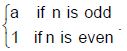• Product of n consecutive numbers is always divisible by n!.

Example 1. If ‘X’ is an even number; Y is an odd number, then which of the following is even?
(a) X2 + Y
(b) X + Y2
(c) X2 + Y2
(d) X2Y2
(e) None of these

• Since X is even, X2 is even.
• Y is odd, Y2 is odd.
• So options (1), (2), (3) are even + odd = odd.
• Option (4) is (even) (odd) = Even.

Example 2. What is the difference between 0.343434....…and 0.2343434…… in fraction form?
(a) 6/55
(b) 6/11
(c) 9/55
(d) 9/13
(e) 5/11

0.343434.....= 34/99 and 0.23434.... = 234 - 2/990 = 232/990
∴ Difference = 34/99 - 232/990 = 108/990 = 6/55

Example 3. How many of the following numbers are divisible by at least 3 distinct prime numbers 231, 750, 288 and 1372?
(a) 0
(b) 1
(c) 2
(d) 3
(e) 4

• 231 = 3 × 7 × 11 (3 prime factors)
• 750 = 2 × 3 × 53 (3 prime factors)
• 288 = 25 × 32 (only 2 prime factors)
• 1372 = 22 × 73 (only 2 prime factors)
• So, only 231 & 750 has 3 prime factors

Example 4. n3 + 6n2 + 11n + 6 (where n is a whole no) is always divisible by
(a) 4
(b) 5
(c) 6
(d) 8
(e) 12

• n3 + 6n2 + 11n + 6 = (n + 1) (n + 2) (n + 3).
• Product of 3 consecutive numbers is always divisible by 3! = 6. (or)Take n = 0, 1, 2, 3 and check it is always divisible by 6.

Example 5. What is the remainder, if 351 × 352 × 353 × - - - - - - - × 356 is divided by 360?
(a) 0
(b) 1
(c) 2
(d) 3
(e) 359

• Since the given is the product of 6 consecutive numbers, it is always divisible by 6! = 720.
• It is divisible by 360 also. So, the remainder will be 0.
The document Introduction: Number System - Notes | Study CSAT Preparation for UPSC CSE - UPSC is a part of the UPSC Course CSAT Preparation for UPSC CSE.
All you need of UPSC at this link: UPSC

## CSAT Preparation for UPSC CSE

72 videos|64 docs|92 tests
 Use Code STAYHOME200 and get INR 200 additional OFF

## CSAT Preparation for UPSC CSE

72 videos|64 docs|92 tests

### How to Prepare for UPSC

Read our guide to prepare for UPSC which is created by Toppers & the best Teachers

Track your progress, build streaks, highlight & save important lessons and more!

,

,

,

,

,

,

,

,

,

,

,

,

,

,

,

,

,

,

,

,

,

;